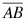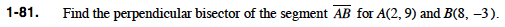### Home > PC > Chapter 1 > Lesson 1.2.2 > Problem1-81

1-81.

Find the perpendicular bisector of the segmentfor A(2, 9) and B(8, −3). Homework Help ✎Find the slope of the line.

Find the midpoint of the line.

Find the slope of the perpendicular line.

Use the point slope form of a line substituting one of the known points and your perpendicular slope.

$m = \frac{9 - (-3)}{8 - 2} = \frac{12}{6} = 2$

$\left ( \frac{8 + 2}{2}, \frac{9 + (-3)}{2} \right ) \left ( \frac{10}{2}, \frac{6}{2} \right ) = (5,3)$

It is the negative reciprocal of the slope of the line:

$-\frac{1}{2}$

$y - 3 = -\frac{1}{2}(\textit{x} - 5)$